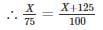Two trains start at the same time from two point P and Q and proceed towards each other at the speed of 75 km/hr and 100 km/hr respectively. When they meet, it is found that first train has travelled 125 km less than the second train. Find the distance between point P and Q.
• a)
1365 km
• b)
540 km
• c)
560 km
• d)
875 km

### Related TestBAGLA LALIT Oct 06, 2019Let the distance travelled by first train till the point they meet be X km. Since the second train travelled 125 Km more than the first one, thus
Distance travelled by second train when they meet = X + 125
We know that, Time = Distance/Speed
We know that both trains travelled for the same time till they meet. Hence,⇒ 100X = 75X + 9375
⇒ X = 375 Km
∴ X + 125 = 500 Km
Hence the distance between the points P and Q = Distance travelled by train A & B
= 375 + 500
= 875 Km

Let the distance travelled by first train till the point they meet be X km. Since the second train travelled 125 Km more than the first one, thusDistance travelled by second train when they meet = X + 125We know that, Time = Distance/SpeedWe know that both trains travelled for the same time till they meet. Hence,⇒ 100X = 75X + 9375⇒ X = 375 Km∴ X + 125 = 500 KmHence the distance between the points P and Q = Distance travelled by train A & B= 375 + 500= 875 Km
Let the distance travelled by first train till the point they meet be X km. Since the second train travelled 125 Km more than the first one, thusDistance travelled by second train when they meet = X + 125We know that, Time = Distance/SpeedWe know that both trains travelled for the same time till they meet. Hence,⇒ 100X = 75X + 9375⇒ X = 375 Km∴ X + 125 = 500 KmHence the distance between the points P and Q = Distance travelled by train A & B= 375 + 500= 875 Km